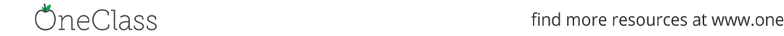Class Notes (1,100,000)
CA (650,000)
UTSG (50,000)
MAT (4,000)
Lecture 1

# MAT247H1 Lecture Notes - Lecture 1: Kingdom Of Sine, Linear Map, Joule

Department
Mathematics
Course Code
MAT247H1
Professor
Fiona T Rahman
Lecture
1

Page:
of 4MAT247 - Problem Set 1 Solution
Problem 1
a) It is straightforward to verify this is an inner product.
b) Again, easily veriﬁed using matrix multiplication.
c) Not an inner product. We have < x, x >=x2
14x2
2, take x1= 5 and
x2= 1, we see that <·,·>is not positive deﬁnite. Hence, not an inner
product.
d) Not an inner product. Take f(x) = x(xi) then < f, f >= 0, but f6= 0,
hence not an inner product.
e) Easy to verify it is an inner product. For condition 4, we use the property
that <·,·>0is an inner product, hence we get positive deﬁniteness.
Problem 2
In this question, to be precise, we need to make sure the deﬁnition is well-
deﬁned. If xspan{v1, . . . , vn}, with vi’s in β(i.e x=Pn
i=1 aivi), then for
any other basis vector wβ, the only way to represent xas an element
of span{v1, . . . , vn, w}is by x=a1v1+. . . +anvn+ 0 ·w, since βis a
basis. Therefore, in computing < x, y >, taking any ﬁnite collection of
basis elements whose span contains both xand ywill give the same answer.
It is then straightforward to verify that we indeed get an inner product, and
in particular, in the case of Vis ﬁnite-dimensional one recovers the standard
inner product.
1
Problem 3
First, convince yourself that we’ve been given an inner product. Let u1=
(1,1,0), u2= (1,0,1), u3= (0,1,1). We apply the Gram-Schmidt algorithm:
take v1=u1, and set
v2=u2< u2, v1>
||v1||2v1= (1,0,1) 3
5(1,1,0) = (2/5,3/5,1)
v3=u3< u3, v1>
||v1||2v1< u3, v2>
||v2||2v2= (0,1,1) 2
5(1,1,0) (1/5)
(55/25)(2/5,3/5,1)
=(4/11,6/11,12/11)
and {v1, v2, v3}form an orthogonal basis. If v= (0,2,1), we can write
v=< v, v1>
||v1||2v1+< v, v2>
||v2||2v2+< v, v3>
||v3||2v3=4
5v1v2+9
4v3
Problem 4
One approach would be to apply the Gram-Schmidt procedure to the basis
{1, x, x2}, which yields an orthonormal basis {1
2,1
2(x1), x2+ 1}. Then, as
in problem 3 (or by eyeball), we can write
x2+ (1 + i)x+i= (x2+1)+(1+i)2(x1
2)+22i(1
2)
Of course, if you chose a diﬀerent basis your answers may vary.
Problem 5
Note that W=span{(1,0,2,1),(0,1,0, i)}, so applying the Gram-Schmidt
procedure to these two vectors (which are clearly independent) and then nor-
malizing yields a basis {1
2(1,0,2,1),1
27(i, 4,2i, 3i)}.
Now W={(x, y, z, w)C4|x+2zw= 0, y +iw = 0}, which is
spanned by (say) (2,0,1,0) and (0,2i, 1,2). Applying Gram-Schmidt
and normalizing gives a basis {1
3(2,0,1,0),1
118 (2/5,2i, 4/5,2)}for
W.
Problem 6
Let (tij ) be the matrix of Twith respect to the basis β={x1, . . . , xn}. For
any v=Pn
i=1 aixiV, we have that
< T (v), T (v)>=< T (
n
X
i=1
aixi), T (
n
X
j=1
ajxj)>=
n
X
i,j=1
aiaj< T (xi), T (xj)>
=
n
X
i,j=1
aiaj< T (xi),
n
X
k=1
tkj xk>
=
n
X
i,j,k=1
aiajtkj < T (xi), xk>= 0
2
since each < T (xi), xj>= 0. So for each vV,T(v) = 0; i.e., Tis the zero
operator.
Problem 7
a) Suppose Vis an n-dimensional complex inner product space, and Tis an
invertible linear operator on V. Let cT(x) = det(x·IdT) be the characteristic
polynomial of T. This is a polynomial of degree nover the complex numbers,
hence it has a zero (Cis an algebraically closed ﬁeld - Appendix D in the
text has one proof of this fact). In other words, there is a λCsuch that
det(λ·Id T) = 0, and so there is some non-zero xVsuch that T(x) = λx.
Applying T1to this expression, we have x=λT 1(x), which implies λ6= 0,
since xis non-zero.
Thus,
< T (x), x >=< λx, x >=λ < x, x >=λ||x||26= 0
since both xand λare non-zero.
b) Now suppose Vis real, and has odd dimension. The characteristic
polynomial cT(x) = det(x·IdT) = xn+. . . is a (real) polynomial in xof degree
n, which is odd, and so it has a (real) zero ( note that limx+cT(x) = +
and limx→−∞ cT(x) = −∞, so being a continuous function cT(x) has to hit
the x-axis somewhere). So, as above, there is some λRand (non-zero)
xVsuch that T(x) = λx. Applying T1, we see that λ6= 0, and that
< T (x), x >=λ||x||26= 0.
c) Now suppose Vis real, and of dimension n= 2m. Changing notation
slightly, let β={x1, . . . xm, y1, . . . ym}be an orthonormal basis for V, and deﬁne
U:VVon basis elements by U(xi) = yi,U(yi) = xi(a linear operator is
clearly determined uniquely by where it sends basis elements). Let T:VVbe
deﬁned by T(xi) = yi,T(yi) = xi. Then TUand UTare both the identity,
so T=U1i.e. Uis invertible. However, for any x=Pm
i=1 aixi+biyiV, we
have
< U(x), x > =<
m
X
i=1
aiU(xi) + biU(yi),
m
X
j=1
ajxj+bjyj>
=<
m
X
i=1
ai(yi) + bi(xi),
m
X
j=1
ajxj+bjyj>
=
m
X
i,j=1
(aiaj< yi, xj>aibj< yi, yj>+biaj< xi, xj>+bibj< xi, yj>)
=
m
X
i=1
(aibi< yi, yi>+biai< xi, xi>)
=
m
X
i=1
(aibi+aibi) = 0
where the fourth and ﬁfth lines follow from the assumption that our basis was
orthonormal.
3